voltage drops, and so forth. "> Useful AC FormulasCustom SearchUSEFUL AC FORMULAS PERIOD TIME (t)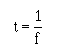FREQUENCY (f)AVERAGE VOLTAGE OR CURRENT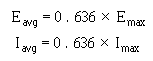EFFECTIVE VALUE OF VOLTAGE OR CURRENT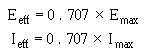MAXIMUM VOLTAGE OR CURRENTOHM'S LAW OF AC CIRCUIT CONTAINING ONLY RESISTANCEL/R TIME CONSTANT (TC)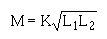TOTAL INDUCTANCE (LT) Series without magnetic couplingTOTAL INDUCTANCE (LT) PARALLEL (No magnetic coupling)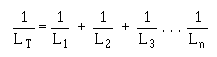CAPACITANCE (C)RC TIME CONSTANT (t)TOTAL CAPACITANCE (CT) SERIES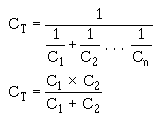TOTAL CAPACITANCE (CT) PARALLELINDUCTIVE REACTANCE (XL)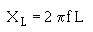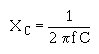IMPEDANCE (Z)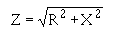OHM'S LAW FOR REACTIVE CIRCUITS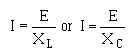OHM'S LAW FOR CIRCUITS CONTAINING RESISTANCE AND REACTANCE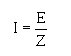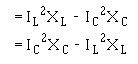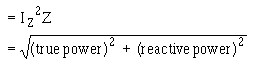POWER FACTOR (PF)VOLTAGE ACROSS THE SECONDARY (Es)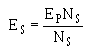VOLTAGE ACROSS THE PRIMARY (Ep)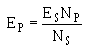CURRENT ACROSS THE SECONDARY (Is)CURRENT ACROSS THE PRIMARY (Ip)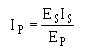TRANSFORMER EFFICIENCY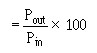TRIGONOMETRIC FUNCTIONS In a right triangle, there are several relationships which always hold true. These relationships pertain to the length of the sides of a right triangle, and the way the lengths are affected by the angles between them. An understanding of these relationships, called trigonometric functions, is essential for solving problems in a-c circuits such as power factor, impedance, voltage drops, and so forth. To be a RIGHT triangle, a triangle must have a "square" corner; one in which there is exactly 90° between two of the sides. Trigonometric functions do not apply to any other type of triangle. This type of triangle is shown in figure V-1. By use of the trigonometric functions, it is possible to determine the UNKNOWN length of one or more sides of a triangle, or the number of degrees in UNKNOWN angles, depending on what is presently known about the triangle. For instance, if the lengths of any two sides are known, the third side and both angles &thetas; (theta) and Φ (phi) may be determined. The triangle may also be solved if the length of any one side and one of the angles (&thetas; or Φ in fig. V-1) are known. Figure V-1. - A right triangle.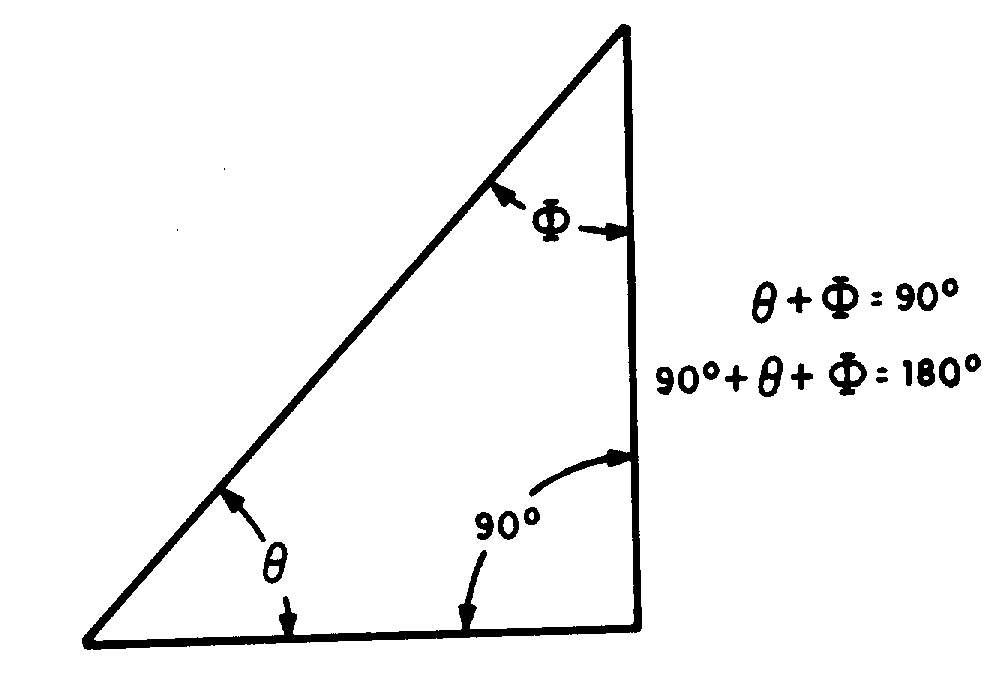The first basic fact of triangles is that IN ANY RIGHT TRIANGLE, THE SUM OF THE THREE ANGLES FORMED INSIDE THE TRIANGLE MUST ALWAYS EQUAL 180°. If one angle is always 90° (a right angle) then the sum of the other two angles must always be 90°.thus, if angle &thetas; is known, Φ may be quickly determined. For instance, if &thetas; is 30°m what is Φ ?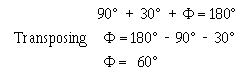Also, if &thetas; is known, Φ may be determined in the same manner. The second basic fact you must understand is that FOR EVERY DIFFERENT COMBINATION OF ANGLES IN A TRIANGLE, THERE IS A DEFINITE RATIO BETWEEN THE LENGTHS OF THE THREE SIDES. Consider the triangle in figure V-2, consisting of the base, side B; the altitude, side A; and the hypotenuse, side C. (The hypotenuse is always the longest side, and is always opposite the 90° angle.) Figure V-2. - A 30° - 60° - 90° triangle.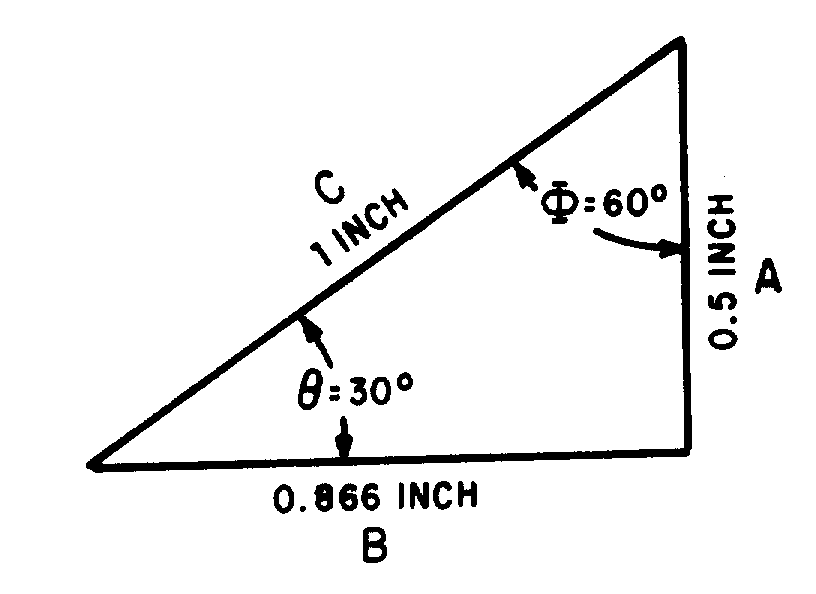If angle &thetas; is 30°, Φ must be 60°. With &thetas; equal to 30°, the ratio of the length of side B to side C is 0.866 to 1. That is, if the hypotenuse is 1 inch long, the side adjacent to &thetas;, side B, is 0.866 inch long. Also, with &thetas; equal to 30°, the ratio of side A to side C is 0.5 to 1. That is, with the hypotenuse 1 inch long, the side opposite to &thetas; (side A) is 0.5 inch long. With &thetas; still at 30°, side A is 0.5774 of the length of B. With the combination of angles given (30°-60°-90°) these are the ONLY ratios of lengths that will "fit" to form a right triangle. Note that three ratios are shown to exist for the given value of &thetas;: the ratio B\C which is always referred to as the COSINE ratio of &thetas;, the ratio A\C, which is always the SINE ratio of &thetas;, and the ratio A\B, which is always the TANGENT ratio of &thetas;. If &thetas; changes, all three ratios change, because the lengths of the sides (base and altitude) change. There is a set of ratios for every increment between 0° and 90°. These angular ratios, or sine, cosine, and tangent functions, are listed for each degree and tenth of degree in a table at the end of this appendix. In this table, the length of the hypotenuse of a triangle is considered fixed. Thus, the ratios of length given refer to the manner in which sides A and B vary with relation to each other and in relation to side C, as angle &thetas; is varied from 0° to 90°. The solution of problems in trigonometry (solution of triangles is much simpler when the table of trigonometric functions is used properly. The most common ways in which it is used will be shown by solving a series of exemplary problems. Problem 1: If the hypotenuse of the triangle (side C) in figure V-3 is 10 inches long, and angle &thetas; is 33°, how long are sides B and A? Figure V-3. - Problem 1.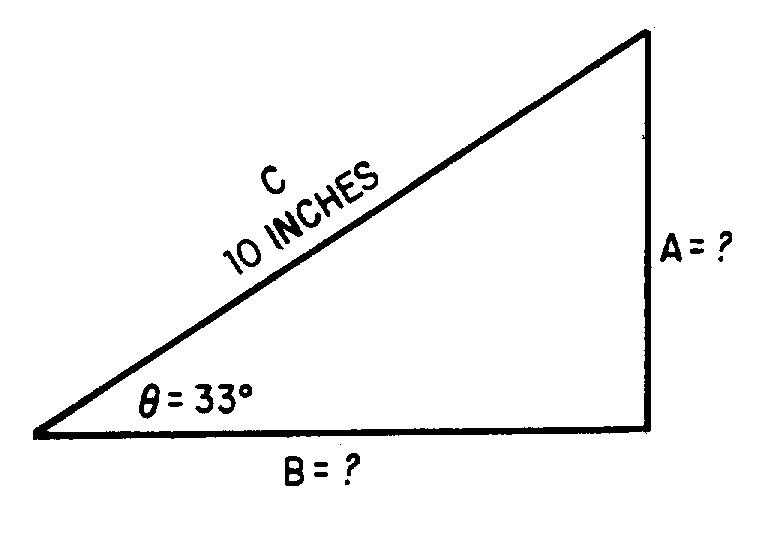Solution: The ratio B/C is the cosine function. By checking the table of functions, You will find that the cosine of 33° is 0.8387. This means that the length of B is 0.8387 the length of side C. If side C is 10 inches long, then side B must be 10 X 0.8387, or 8.387 inches in length. To determine the length of side A, use the sine function, the ratio A\C. Again consulting the table of functions, you will find that the sine of 33° is 0.5446. Thus, side A must be 10 X 0.5446, or 5.446 inches in length. Problem 2: The triangle in figure V-4 has a base 74.2 feet long, and hypotenuse 100 feet long. What is &thetas;, and how long is side A? Figure V4. - Problem 2.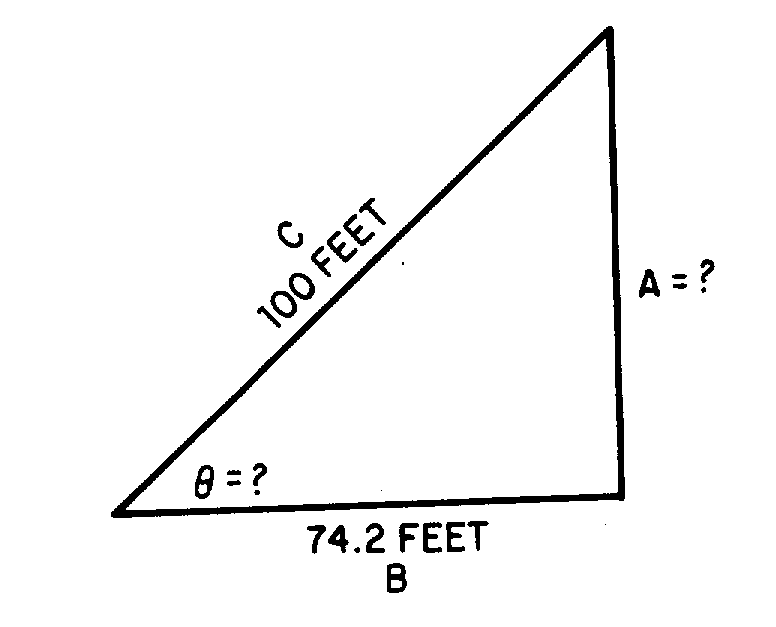Solution: When no angles are given, you Must always solve for a known angle first. The ratio B\C is the cosine of the unknown angle &thetas;; therefore 74.2/100 or 0.742, is the cosine of the unknown angle. Locating 0.742 as a cosine value in the table, you find that it is the cosine of 42.1°. That is, &thetas; = 42.1°. With &thetas; known, side A is solved for by use of the sine ratio A/C. The sine of 42.1°, according to the table, is 0.6704. Therefore, side A is 100 X 0.6704, or 67.04 feet long. Problem 3: In the triangle in figure V-5, the base is 3 units long, and the altitude is 4 units. What is &thetas;, and how long is the hypotenuse? Solution: With the information given, the tangent of &thetas; may be determined. Tan &thgr; = A/B = 4/3 = 1.33. Figure V-5. - Problem 3.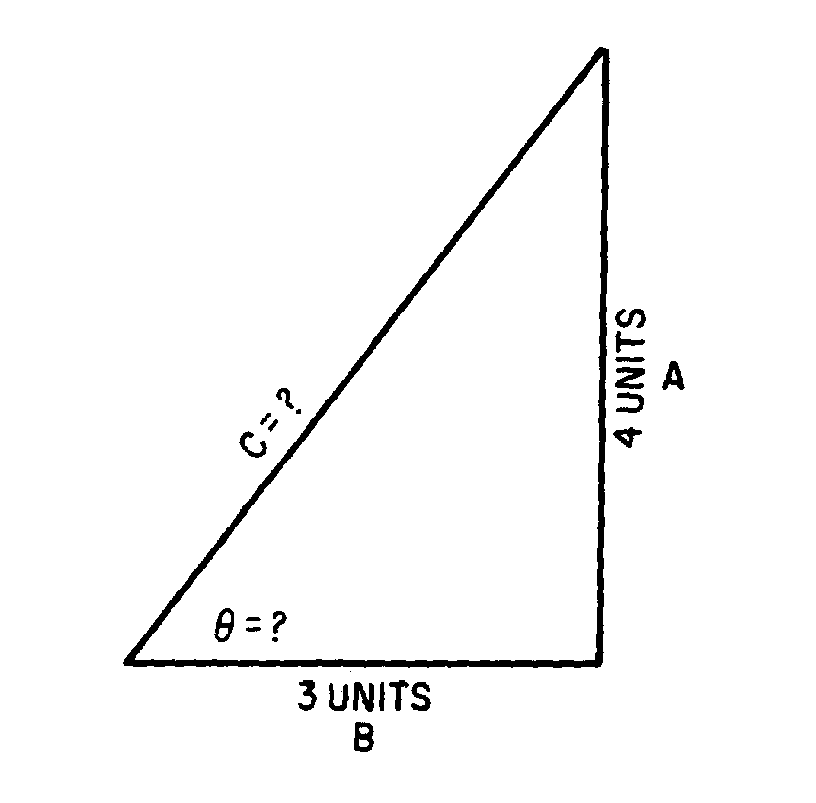Locating the value 1.33 as a tangent value in the table of functions, you find it to be the tangent of 53.1°. Therefore, &thetas; = 53.1°. Once &thetas; is known, either the sine or cosine ratio may be used to determine the length of the hypotenuse. The cosine of 53.1° is 0.6004. This indicates that the base of 3 units is 0.6004, the length of the hypotenuse. Therefore, the hypotenuse is 3/0.6004, or 5 units in length. Using the sine ratio, the hypotenuse is 4/0.7997, or 5 units in length. In the foregoing explanations and problems, the sides of triangles were given in inches, feet, and units. In applying trigonometry to a-c circuit problems, these units of measure will be replaced by such values given in ohms, amperes, volts, and watts. Angle &thetas; will be the phase angle between (source) voltage and circuit current. However, the solution of these a-c problems is accomplished in exactly the same manner as the foregoing problems. Only the units and some terminology are changed.Integrated Publishing, Inc. - A (SDVOSB) Service Disabled Veteran Owned Small Business